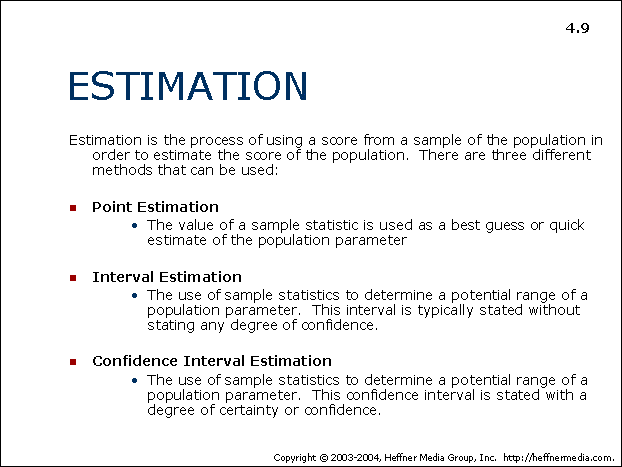# 09 – Estimation## ESTIMATION

Estimation is the process of using a score from a sample of the population in order to estimate the score of the population. There are three different methods that can be used:

• Point Estimation
• The value of a sample statistic is used as a best guess or quick estimate of the population parameter
• Interval Estimation
• The use of sample statistics to determine a potential range of a population parameter. This interval is typically stated without stating any degree of confidence.
• Confidence Interval Estimation
• The use of sample statistics to determine a potential range of a population parameter. This confidence interval is stated with a degree of certainty or confidence.